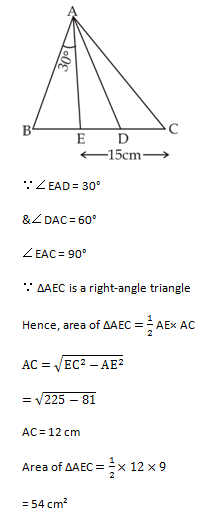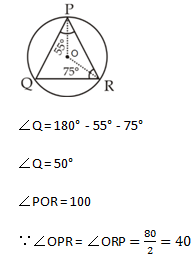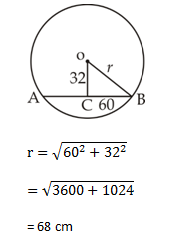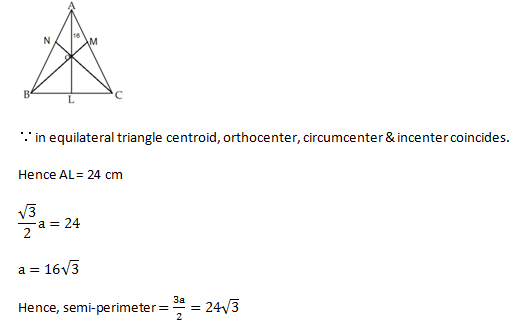# SSC Quantitative Aptitude Questions (Day-62)

Dear Aspirants, you can find the Quantitative Aptitude questions with detailed explanations for the SSC exams. Nowadays the competitive level of the exam has been increasing consistently. Due to the great demand for the government job, the level of the toughness reached greater. Candidates have to enhance the preparation process in order to drive in the right path. It doesn’t need to clear the prescribed cutoff. You must have to score good marks more than the cut off marks to get into the final provisional list. Here we have updating the Quantitative Aptitude questions with detailed explanations on a daily basis. You can practice with us and measure your level of preparation. According to that you can sculpt yourself in a proper way. SSC aspirants kindly make use of it and grab your success in your career.

Start Quiz

1) If x – y = 3, then what is the value of x3 – y3 – 9xy?

(a) 3

(b) 9

(c) 18

(d) 27

2) If x2 – 9x + 1 = 0, then what is the value of x3+
1/x3 ?

(a) 54

(b) 108

(c) 702

(d) 810

3)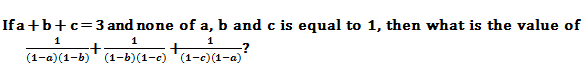(a) 0

(b) 1

(c) 3

(d) 6

4)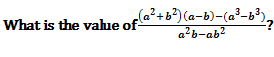(a) 0

(b) 1

(c) -1

(d) 3

5) What is the simpliﬁed value of (x128 + 1)(x32 + 1)(x64 + 1)(x16 + 1)(x8 + 1)(x4 + 1)(x2 + 1)(x + 1)?

(a) x256 – 1

(b)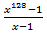(c)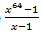(d)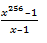6) DEF is an isosceles triangle with such that DE = DF = 60 cm and EF = 96 cm. DG is a median to base EF. What is the length (in cm) of DG?

(a) 22

(b) 36

(c) 24

(d) 32

7) In ΔABC, AD and AE are bisectors of ∠BAC and ∠BAD respectively. If ∠BAE = 30°, AE = 9 cm and EC = 15 cm, what is the area (in cm2) of ΔAEC?

(a) 36

(b) 54

(c) 72

(d) 216

8) Circum-centre of ΔPQR is O. If ∠QPR = 55° and ∠QRP = 75°, what is the value (in degrees) of ∠OPR?

(a) 45

(b) 40

(c) 65

(d) 70

9) A chord of length 120 cm is at a distance of 32 cm from the centre of a circle. What is the radius (in cm) of the circle?

(a) 96

(b) 34

(c) 72

(d) 68

10) ABC is a equilateral triangle. O is the point of intersection of altitudes AL, BM and CN. If OA = 16 cm, then what is the semi-perimeter (in cm) of the triangle ABC?

(a) 8√3

(b) 12√3

(c) 16√3

(d) 24√3

x – y = 3

On doing cube of both sides,

x³ – y³ – 3xy (x – y) = 27

x³ – y² – 3xy.3 = 27

x³ – y³ – 9xy = 27

x²  –  9x + 1 = 0

x +  1/x + = 9

Hence, x3 + 1/x3 =729 – 27

= 702

Assume b = c =0 & a = 3

Then,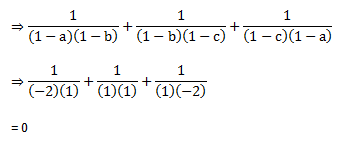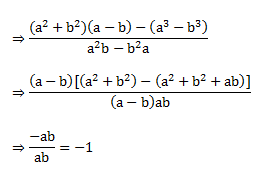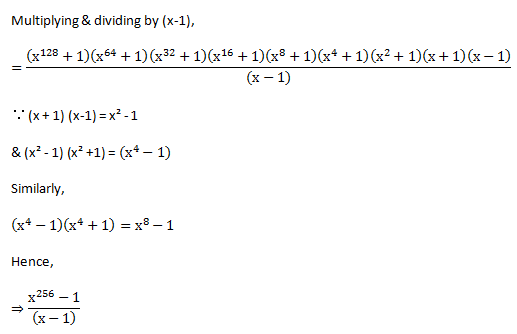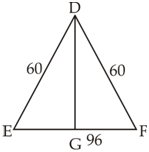ByApollonius theorem,

DE² + DF² = 2(48² + DG²)

3600 = 48² + DG²

3600 = 2304 = DG²

DG = 36 cm